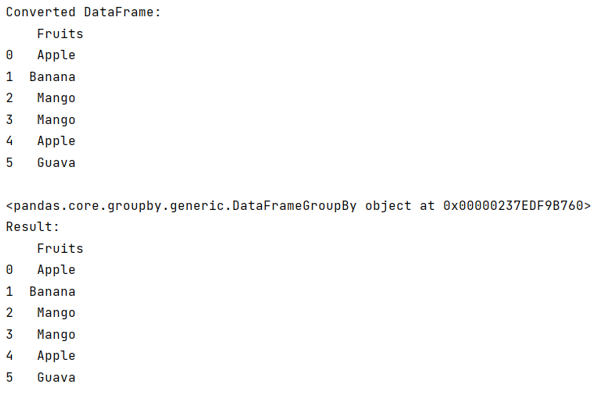# How to group a series by values in pandas?

Given a Pandas DataFrame, we have to group a series by values. By Pranit Sharma Last updated : September 25, 2023

Pandas is a special tool that allows us to perform complex manipulations of data effectively and efficiently. Inside pandas, we mostly deal with a dataset in the form of DataFrame. DataFrames are 2-dimensional data structures in pandas. DataFrames consist of rows, columns, and data.

A Series contains a single list that can store heterogeneous types of data, because of this, a series is also considered a 1-dimensional data structure. When we analyze a series, each value can be considered as a separate row of a single column.

## Problem statement

Given a Pandas DataFrame, we have to group a series by values.

## Grouping a series by values in pandas?

To group a series by values in pandas, we will use first convert the series into a dataframe, and then we will use pandas.DataFrame.groupby() method. This method is used to group the data inside DataFrame based on the condition passed inside it as a parameter. It works on a split and group basis. It splits the data and then combines them in the form of a series or any other sequence.

Note

To work with pandas, we need to import pandas package first, below is the syntax:

```import pandas as pd
```

Let us understand with the help of an example,

## Python program to group a series by values

```# Importing pandas package
import pandas as pd

# Creating a series
ser = pd.Series(['Apple','Banana','Mango','Mango','Apple','Guava'])

# Converting series into dataframe
df=pd.DataFrame(ser,columns=['Fruits'])

# Dispaly DataFrame
print("Converted DataFrame:\n",df,"\n")

# Applying groupby
df = df.groupby(['Fruits'])

# Display result
print("Result:\n",df)
```

### Output

The output of the above program is: